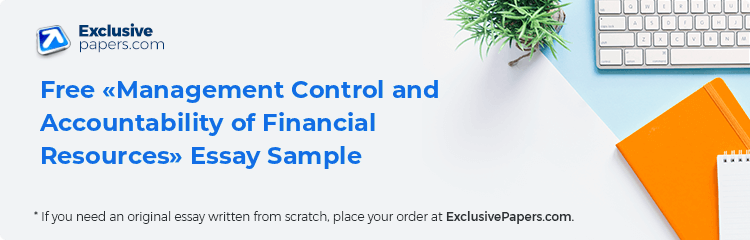## Question 1

a) Break-even point = fixed cost/selling price - variable cost

Fixed cost = £15000

Price = £5

Variable cost per item:

Components =£3.00

Packaging = £0.50

Break point = 15000/ (5-(3+0.5))

= 15000/1.5

= 10,000.

To break even, Bob has to sell 10,000 test boxes.

b) For the year, the number of test boxes to be sold is 10,000 without profit.

To make a profit of £5000 for the year, the total sales will cost (10,000*5) + £5000

= £550000

Each test box is equivalent to £5. Therefore, £55000 is equivalent to 15714 boxes.

c) Sales

Gross sales                             (15000*5)                               = £75000

Less cost of goods sold         (3.5*15000)                            =£52500

Net sales                                                                               =£22500

## Expenses

Rent                                                                                      =£15000

Total Expenses                                                                      =£15000

Net Profit                                                                              =£7500

d) Price =£4.5

New price of components = 3.0-2.75

=£0.25

Target profit = £5000

Break point = 15000/(4.5-(0.25+0.5)

= 4000

4000*4.5)+ £5000

Bob has to sell 5111boxes to achieve his target profit of £5000                                                                                                                                                                                                                                                       e)  Circumstances that may lead to Bob selling the items at a price lower than the full cost is when the number of customers has increased beyond the expected one in a given time period.

f) Fixed cost refers to costs which remain fixed up to a certain range of capacity no matter what amount of goods is produced within that capacity range. Variable cost means costs that alter with a change in the number of product units produced.

## Question 2

a)       Recognized elements to published financial reports and statements are:

• Assets
• Liabilities
• Capital
• Net income

b)       Users of published financial reports are:

Present and future investors

## Financial analysts

i. Cost of sales

Cost of sales = purchases +expenses

= (40+125)*1000

=£165000

ii. Gross profit = £ (1257-(40+165))*1000

= £ (1257 – 205)*1000

=£1052000

iii. Net profit

£1052000-£125000=£927000

iv. Gross profit margin

v. Stock turnover ratio

Inventory turnover ratio = 653.5

Cost of goods sold = 165000

Turnover ratio = 1£65000/653.5=£ 252.487

vi. Creditor turnover ratio (days)

Creditors Turnover = COGS / Creditors (A/c Payables) .

165,000/45000=3.67

vii. Debtor turnover ratio (days)

net credit sales / average debtors

45,000/120000=0.375

## Question 3

a)      Benefits of budgeting are:

• Integrating and coordinating activities.
• Provision of communication devices to the employees of an organization.
•  Promoting continuous improvement.
• Guides performance.

b) The main elements of a budget comprise the money source, the known amount of money that must be spent, amount left over for daily living and, lastly, the records of what is actually earned and spent monthly.

i. Incremental budgeting

This is a budget that has been prepared with reference to the previous period’s budget as a basis of incremental amounts added to the new budget period.

ii. Zero-based budgeting

This is a budgeting method in which the entire expenses must be warranted for each additional phase.

iii. Flexible budgeting

This is a type of budget which shows expected revenue and costs at a variety of different activity levels.

Negative balance shows cash deficiency to the management of the company.

## Question 4

a)

1. Risk refers to the probability of damage, injury, liability, loss or any other negative occurrences caused by internal or external vulnerabilities.
2.   Risk management refers to the process involved in the identification and either mitigation or acceptance of uncertainty in investment decision making.
3. The main steps of managing risks are:
• Identifying the foreseeable hazards in the workplace which can potentially affect everyone at the work place.
• Assessing the amount of risk from the hazard.
• Controlling the hazard and, in some cases, eliminating the risk completely.
• Reviewing risk assessment to monitor and improve measure put in place and finding safe ways of carrying out activities.

b) Estimated unit cost of production:

=20000+11500+5350=£36850

a) Materials cost 20% higher than expected.

The new cost of material is 120%*20000=£24000

Therefore, new estimated unit cost is 24000+11500+5350=£40850

b)  10% higher cost of labor: 110%*11500=£12650

New unit cost is 20,000+12650+5350 = £38000

c)   Expenses are 20% higher.

New expenses = 20%*5350= £6420

New unit cost is 20000+11500+6420=£37920

d) Selling price being reduced by 10%

New selling price will be 90%*50000=45,000

c) The risk of making loss is high since a slight increase in the cost of a single item significantly increases the estimated cost of production.

## Question 5

a)Cost of Capital = 10% *100000

=£ 10000

Depreciation = (initial value – Residual value)/useful life

= (100000 – 10000)/5

=£18000

b)Cash flow/[1+discount rate)^year

Present value of each cash flow is 22727.27, 28925.62, 30052.59, 34150.67, and 31046.07

Total preset value = 952713.7

NPV = £100000 - £ 146902.2 = -852714

 1st 50000 0.91 2 60379.2 2nd 50000 0.83 3 87445.1 3rd 40000 0.75 4 126420 4th 30000 0.68 5 206337 5th 20000 0.62 6 352111 Total 832692

c) When Discount cash flow method is used, Project B is recommended.

d) Provision of discount factors in two decimal places greatly affects the unit price of an item.

Silver member

5% OFF

Gold member

10% OFF

Platinum member

15% OFF

What Our Customers Say

Get 15%OFF   your first custom essay order Use discount code first15